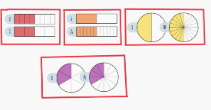# Equivalent Fractions

Computational Thinker### Overview​

The Math Learning Center is an app and online platform that allows students to use manipulatives virtually. In this activity, students will show equivalent fractions using lines and shapes.

Students will:

• Be able to make four equivalent fractions with lines and shapes.

Vocabulary Words:

1. Fraction: A fraction is a part of a whole. A common fraction is made up of a numerator and a denominator. The numerator is shown on top of a line and is the number of parts of the whole. The denominator is shown below the line and is the number of parts by which the whole has been divided.

2. Manipulative: A manipulative is an object which is designed so that a learner can perceive some mathematical concept by manipulating it, hence its name

To prepare for this lesson:

• Teachers will need to have the app or website for virtual manipulatives available to students.

Note: The Math Learning Center has many free apps available for teachers and students. For more information, visit the Math Learning Center

See Accommodations Page and Charts on the 21things4students.net site in the Teacher Resources.

Directions for this activity:

1. Teach students about fractions as parts of a whole. Students should have some prior knowledge of fractions.

2. Take the students to the Fraction website

3. Explain to students that fractions are equivalent when they are the same part of a whole. Example:4. As you show each equivalent fraction, have students help tell you why the fractions are equivalent.

5. Use the annotate feature to label equivalent fractions.

6. Independent Work: Students take what they have learned about fractions and use the app or website to demonstrate 4 equivalent fractions.

7. Students show teacher their work as they finish.

Note: Early finishers may screenshot their work and save it to their computer or upload to an electronic portfolio like SeeSaw.

Different options for assessing the students:

• Observations
• Check for understanding
• Teachers see what students have done through observation. They can intervene if students are struggling with equivalency.

MITECS: Michigan adopted the "ISTE Standards for Students" called MITECS (Michigan Integrated Technology Competencies for Students) in 2018.

Computational Thinker
5c. Students break problems into component parts, extract key information, and develop descriptive models to understand complex systems or facilitate problem-solving.